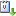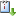##### Personal tools
You are here: Home / 2011 / RISC Colloquium

# RISC Colloquium

Prof. Dr. Joachim von zur Gathen: Census of Polynomials
When Dec 05, 2011 from 01:30 PM to 02:30 PM Seminar RoomvCaliCal

The Prime Number Theorem and a well-known result of Gauss count (approximately or exactly) the number of prime (or irreducible) elements in $\mathbb{Z}$ or $\mathbb{F}_q[x]$. Leonard Carlitz, Stephen Cohen, and others considered multivariate polynomials. We now have an exact formula for their number, and similarly for squareful and relatively irreducible (irreducible and not absolutely irreducible) polynomials, and approximations for the decomposable ones.
This talk reports results on univariate decomposable polynomials $f = g \circ h = g(h) \in \mathbb{F}_q[x]$. The TAME CASE, where the characteristic $p$ of $\mathbb{F}_q$ does not divide $n = \deg f$, is fairly well-understood, and we obtain closely matching upper and lower on the number of decomposable polynomials. In the opposite WILD CASE, the bounds are less satisfactory.
The core of this talk deals with the easiest instance of the wild case, where $n = p^2$. We may assume $g$ and $h$ to have degree $p$ and to be monic and original, that is, with constant coefficient $0$.
Besides the trivial case of $p$th power, the additive polynomials $f=x^{p^2} + ax^p + bx$ are of interest, but we exclude them from this talk.
Any $(g,h)$ yields a decomposable $f = g \circ h$,  and the crux of the matter is to count the number of COLLISIONS, where different $(g,h)$ yield the same $f$. Abhyankar introduced the PROJECTIVE POLYNOMIAL $\psi= y^{p+1}-uy+u$. There is an intimate connection between collisions and the roots of $\psi$. As an example, suppose that $l \mid p-1$, $m=(p-1)/l$ and $t \in \mathbb{F}_q^\times$ is a root of $\psi$. Then
$$f = x(x^{l(p+1)}-ux^{l}+u) = (x(x^{l}-ut^{-1})) \circ (x(x^{l}-t)^{m}) = g \circ h.$$
While $g$ and $h$ depend on $t$, $f$ does not. Thus two distinct roots of $\psi$ yield a collision.
There is another, similar, type of collision from different roots of $\psi$. The main result is that these are all possibilities.
Work in progress is to simplify the current proof of this result which relies on the ramification theory of function fields, and to determine exactly the number of such collisions.
Joint work with: Raoul Blankertz, Mark Giesbrecht, Alfredo Viola, Konstantin Ziegler.

« February 2020 »
February
MoTuWeThFrSaSu
12
3456789
10111213141516
17181920212223
242526272829
Upcoming Events
RISC Forum Mar 02, 2020 01:30 PM - 01:45 PM
RISC Forum Mar 09, 2020 01:30 PM - 02:15 PM
RISC Forum Mar 16, 2020 01:30 PM - 01:55 PM
RISC Forum Mar 23, 2020 01:30 PM - 01:45 PM
RISC Forum Mar 30, 2020 01:30 PM - 02:30 PM
Previous events…
Upcoming events…